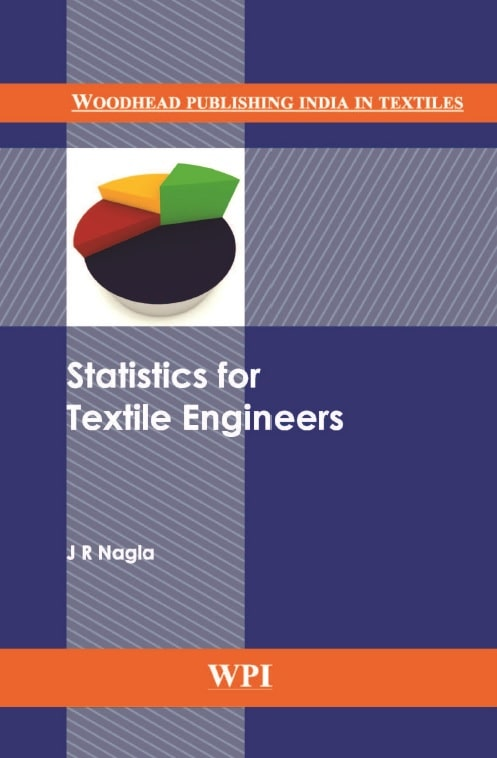# Statistics for Textile Engineers | J R Nagla

Statistics for Textile Engineers
by J. R. NaglaContents

Preface xiii
1 Introduction and need of statistics 1
1.1 Introduction 1
1.2 Nature of textile industry today 1
1.3 Need for SQC techniques 2
2 Statistics and basic concepts 5
2.1 Introduction of statistics 5
2.2 Methods of data collection 7
3 Classification and graphical representations 9
3.1 Introduction 9
3.2 Frequency distribution table 10
3.3 Cumulative frequency distribution table 16
3.4 Graphical representations of the frequency distribution 18
3.5 Graphical representations of the cumulative frequency distribution (Ogive Curve) 20
3.6 Exercise 22
4 Measures of central tendency 25
4.1 Introduction of the central tendency 25
4.2 Measure of central tendency 25
4.3 Arithmetic mean 25
4.4 Computation of AM for the frequency distribution 26
4.5 Median 31
4.6 Computation of median 31
4.7 Graphical determination of median 32
4.8 Mode 35
4.9 Determination of mode 35
4.10 Determination of mode graphically 36
4.11 Exercise 38
5 Partition values 40
5.1 Introduction 40
5.2 Quartiles 40
5.3 Deciles 45
5.4 Percentiles 50
5.5 Exercise 54
6 Measures of dispersion 56
6.1 Introduction of the dispersion 56
6.2 Range 56
6.3 Quartile deviation 58
6.4 Mean deviation 61
6.5 Standard deviation 65
6.6 Relative measures of dispersion 72
6.7 Exercise 76
7 Skewness and kurtosis 80
7.1 Introduction 80
7.2 Skewness 80
7.3 Symmetric frequency distribution 80
7.4 Skewed frequency distribution 81
7.5 Measures of skewness 82
7.6 Interpretation of the coefficient of skewness 83
7.7 Kurtosis 85
7.8 Measures of kurtosis 86
7.9 Exercise 88
8 Correlation and regression 90
8.1 Introduction 90
8.2 Bivariate data 90
8.3 Correlation analysis 90
8.4 Coefficient of determination 93
8.5 Spearman’s rank correlation coefficient 95
8.6 Regression analysis 100
8.7 Exercise 108
9 Multivariate analysis 111
9.1 Introduction 111
9.2 Multivariate data 111
9.3 Multiple correlation analysis 111
9.4 Multiple regression analysis 114
9.5 Exercise 117
10 Probability 119
10.1 Introduction 119
10.2 Basic concepts 119
10.3 Definition of the probability (classical approach) 122
10.4 Laws of the probability 122
10.5 Results of permutation and combination 123
10.6 Exercise 129
11 Probability distributions 131
11.1 Basic concepts 131
11.2 Probability distribution of a random variable 132
11.3 Some properties of the random variable and its probability distribution 136
11.4 Some standard probability distributions 138
11.5 Exercise 138
12 Standard discrete probability distributions 140
12.1 Binomial probability distribution 140
12.2. Poisson probability distribution 143
12.3 Poisson approximation to the binomial distribution 146
12.4 Fitting of binomial and Poisson probability distributions 147
12.5 Exercise 151
13 Standard continuous probability distributions 154
13.1 Normal problem distribution 154
13.2 Standard normal variable and standard normal probability distribution 157
13.3 Chi-square probability distribution (χ2 distribution) 162
13.4 Student’s t-probability distribution 164
13.5 F-probability distribution 166
13.6 Exercise 168
14 Testing of hypothesis 170
14.1 Introduction 170
14.2 Large sample tests (Z-tests) 172
14.3 Small sample tests 185
14.4 Exercise 216
15 Estimation 220
15.1 Introduction 220
15.2 Point estimation 220
15.3 Interval estimation (confidence interval) 222
15.4 Exercise 229
16 Analysis of variance 231
16.1 Introduction 231
16.2 One-way analysis of variance 232
16.3 Two-way analysis of variance 238
16.4 Exercise 249
17 Design of experiments 253
17.1 Introduction 253
17.2. Completely randomized design 254
17.3. Randomized block design 255
17.4. Latin square design (LSD) 257
17.5 Factorial experiments 262
17.6 Exercise 274
18 Statistical quality control 278
18.1 Introduction 278
18.2 Process control 279
18.3 Control chart 279
18.4 Interpretation of control chart 280
18.5 Specification limits 281
18.6 X chart 281
18.7 R-Chart 282
18.8 np-Chart 284
18.9 p-Chart 285
18.10 C-chart 288
18.11 Lot control 290
18.12 Some basic concepts related to rectifying single sampling plan 291
18.13 Exercise 294
Area under standard normal curve from Z = 0 to Z = z 296
tn,∝/2values for t-distribution 298
χ2n,∝ values for χ2-distribution 300
Fm,n, 0.05 values for F-distribution 302
Fm,n, 0.01 values for F-distribution 304
Statistical constants for control charts 306
Index 308

Preface
The inspiration of writing this book is the result of great need from textile engineering students, textile engineers and textile professionals working in the textile industries for the book on statistics in simple and easy language, which will guide and help them to use different statistical methods in decision making.

I hope this book will meet all the needs of textile professionals and textile industries in their decision making as it discusses everything related to statistics right from basic data collection to up to design of experiments with suitable illustrations. Though this book is written for textile-related people only, it is also useful for engineers or researchers of any discipline. The statistical tables of the book are created using Microsoft Excel functions.

I am thankful to Shri. K. Venkatrayan (BITRA Mumbai) for his guidance in writing this book. I am very much thankful to Professor S. D. Mahajan (TEI Ichalkaranji) for writing the introductory chapter, also editing and proof reading the book. I am thankful to my student Raghav Bhala for his help in checking calculations. I am very much thankful to our principal

Dr. P. V. Kadole and the management of DKTE Society for their support and help in completing this book. Finally, I am thankful to those who have inspired and helped me directly or indirectly in writing this book. I will be happy if the readers point out mistakes and give feedback about the book.

J. R. Nagla

This book is US\$10
To get free sample pages OR Buy this book
Send email: [email protected]